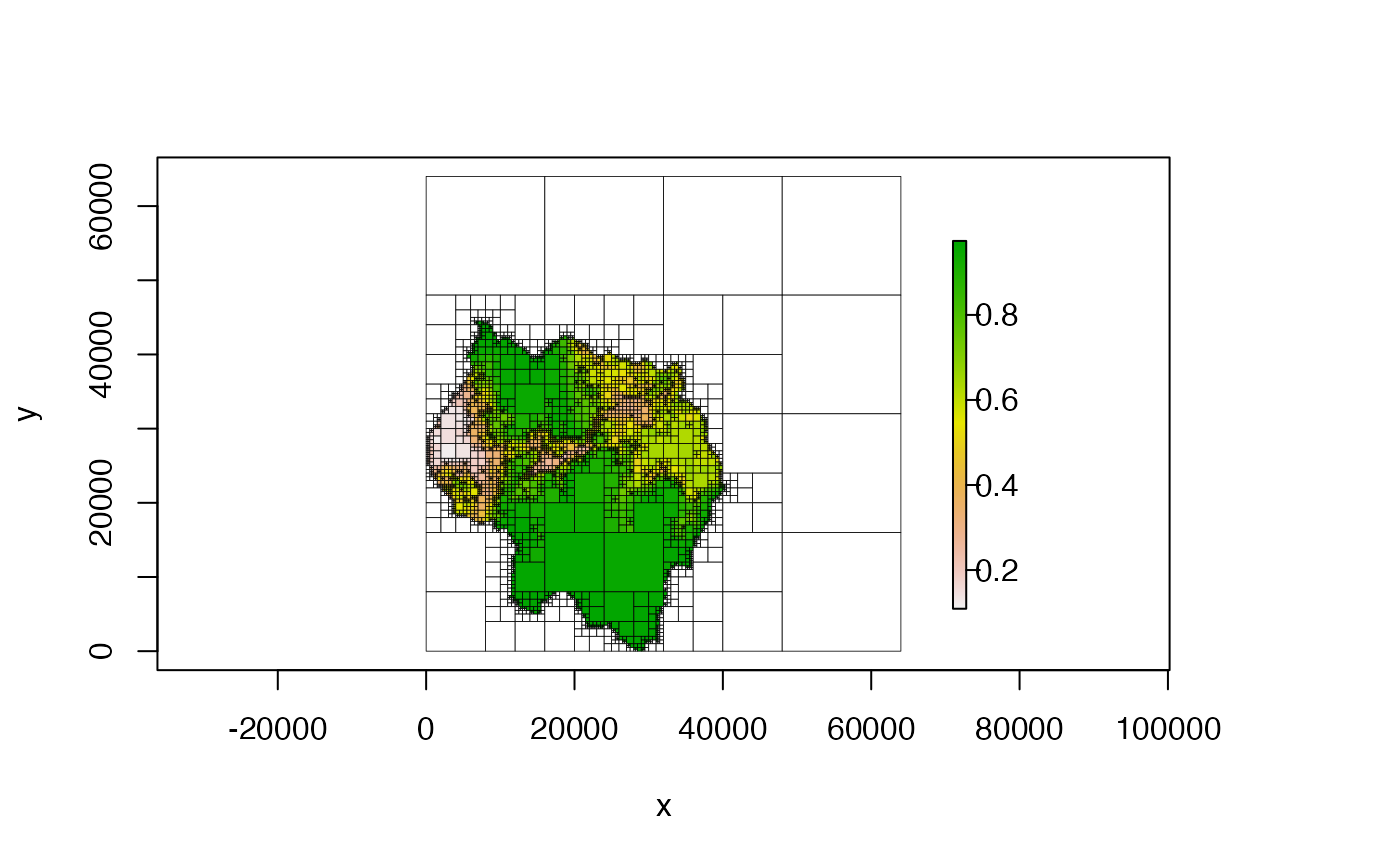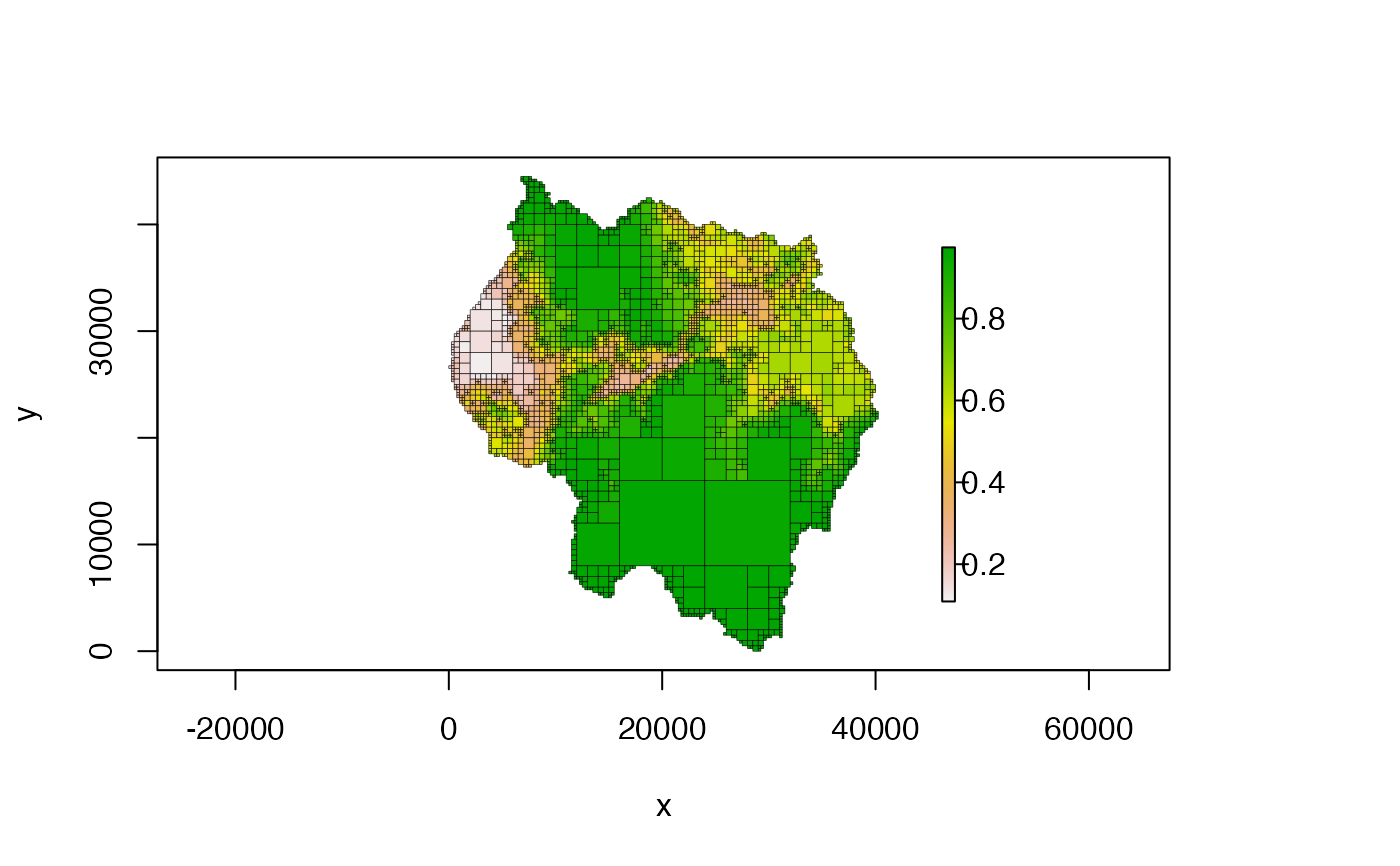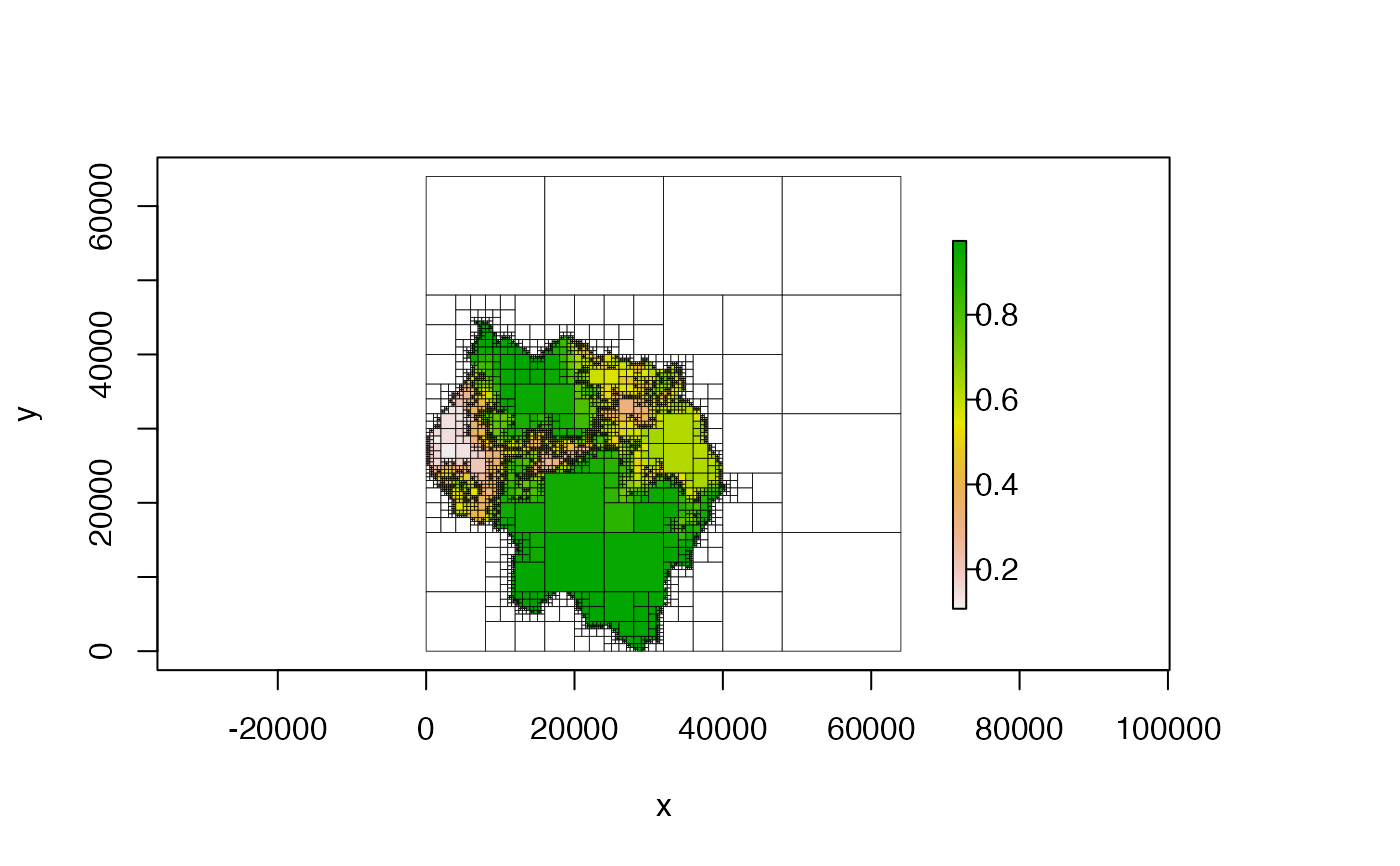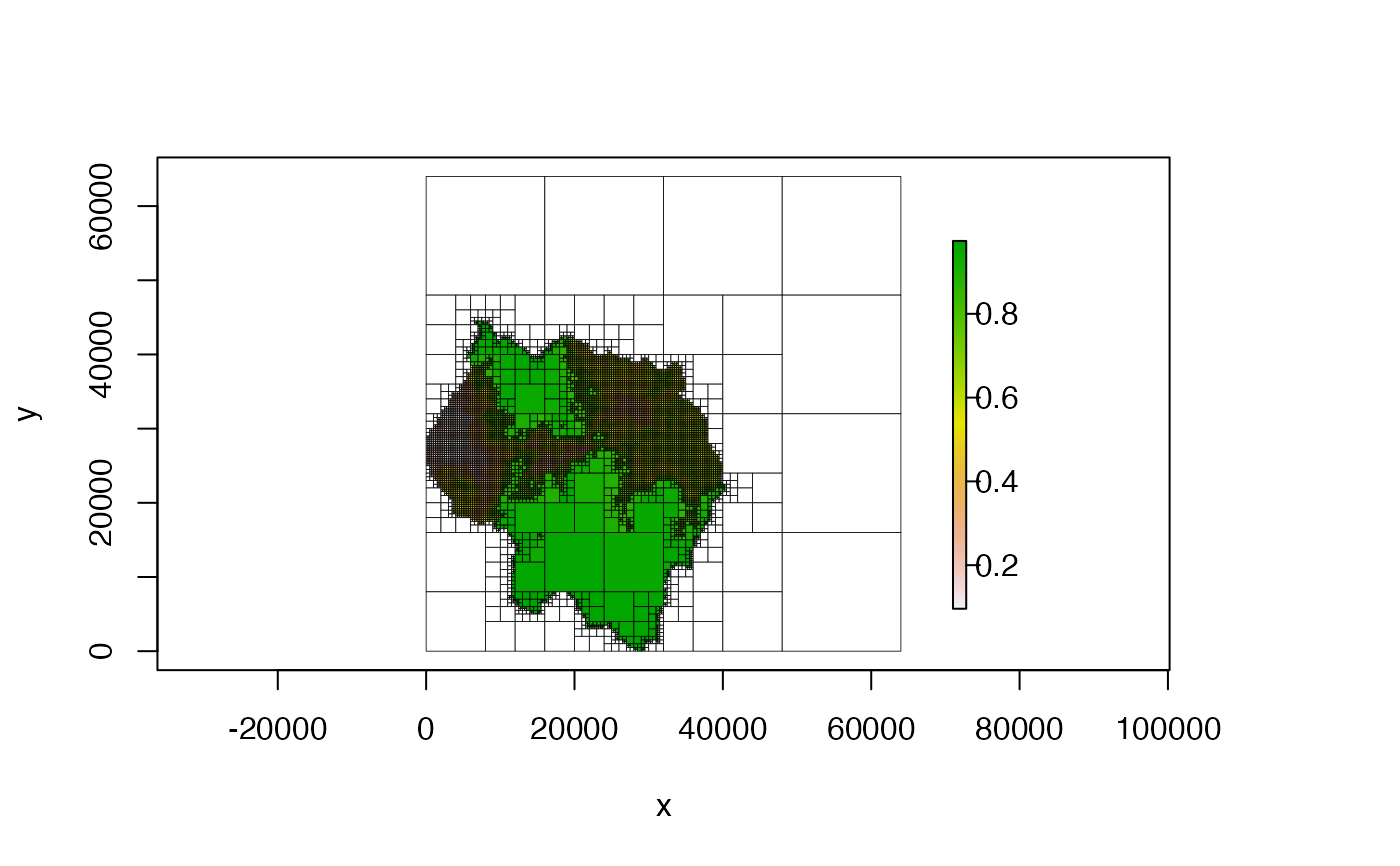Creates a Quadtree from a RasterLayer or a matrix.

# S4 method for ANY
x,
split_threshold = NULL,
split_method = "range",
split_fun = NULL,
split_args = list(),
split_if_any_na = TRUE,
split_if_all_na = FALSE,
combine_method = "mean",
combine_fun = NULL,
combine_args = list(),
max_cell_length = NULL,
min_cell_length = NULL,
resample_n_side = NULL,
extent = NULL,
proj4string = NULL,
)

## Arguments

x

a RasterLayer or a matrix. If x is a matrix, the extent and proj4string parameters can be used to set the extent and projection of the quadtree. If x is a RasterLayer, the extent and projection are derived from the raster.

split_threshold

numeric; the threshold value used by the split method (specified by split_method) to decide whether to split a quadrant. If the value for a quadrant is greater than this value, it is split into its four child cells. If split_method is "custom", this parameter is ignored.

split_method

character; one of "range" (the default), "sd" (standard deviation), "cv" (coefficient of variation) or "custom". Determines the method used for calculating the value used to determine whether or not to split a quadrant (this calculated value is compared with split_threshold to decide whether to split a cell). If "custom", a function must be supplied to split_fun. See 'Details' for more.

split_fun

function; function used on each quadrant to decide whether or not to split the quadrant. Only used when split_method is "custom". Must take two arguments, vals (a numeric vector of the cell values in a quadrant) and args (a named list of arguments used within the function), and must output TRUE if the quadrant is to be split and FALSE otherwise. It must be able to handle NA values - if NA is ever returned, an error will occur.

split_args

list; named list that contains the arguments needed by split_fun. This list is given to the args parameter of split_fun.

split_if_any_na

boolean; if TRUE (the default), a quadrant is automatically split if any of the values within the quadrant are NA.

split_if_all_na

boolean; if FALSE (the default), a quadrant that contains only NA values is not split. If TRUE, quadrants that contain all NA values are split to the smallest possible cell size.

combine_method

character; one of "mean", "median", "min", "max", or "custom". Determines the method used for aggregating the values of multiple cells into a single value for a larger, aggregated cell. Default is "mean". If "custom", a function must be supplied to combine_fun.

combine_fun

function; function used to calculate the value of a quadrant. Only used when combine_method is "custom". Must take two arguments, vals (a numeric vector of the cell values in a quadrant) and args (a named list of arguments used within the function), and must output a single numeric value, which will be used as the cell value.

combine_args

list; named list that contains the arguments needed by combine_fun. This list is given to the args parameter of combine_fun.

max_cell_length

numeric; the maximum side length allowed for a quadtree cell. Any quadrants larger than max_cell_length will automatically be split. If NULL (the default) no restrictions are placed on the maximum cell length.

min_cell_length

numeric; the minimum side length allowed for a quadtree cell. A quadrant will not be split if its children would be smaller than min_cell_length. If NULL (the default) no restrictions are placed on the minimum cell length.

character; one of "expand" (the default), "resample", or "none". Specifies the method used to adjust x so that its dimensions are suitable for quadtree creation (i.e. square and with the number of cells in each direction being a power of 2). See 'Details' for more on the two methods of adjustment.

resample_n_side

integer; if adj_type is 'resample', this number is used to determine the dimensions to resample the raster to.

boolean; only applicable if adj_type is 'resample'. If TRUE (the default), NAs are added to the shorter side of the raster to make it square before resampling. This ensures that the cells of the resulting quadtree will be square. If FALSE, no NAs are added - the cells in the quadtree will not be square.

extent

Extent object or else a four-element numeric vector describing the extent of the data (in this order: xmin, xmax, ymin, ymax). Only used when x is a matrix - this parameter is ignored if x is a raster since the extent is derived directly from the raster. If no value is provided and x is a matrix, the extent is assumed to be c(0,ncol(x),0,nrow(x)).

proj4string

character; proj4string describing the projection of the data. Only used when x is a matrix - this parameter is ignored if x is a raster since the proj4string of the raster is automatically used. If no value is provided and x is a matrix, the proj4string of the quadtree is set to NA.

Quadtree; if provided, the new quadtree will be created so that it has the exact same structure as the template quadtree. Thus, no split function is used because the decision about whether to split is pre-determined by the template quadtree. The raster used to create the template quadtree should have the exact same extent and dimensions as x. If template_quadtree is non-NULL, all split_* parameters are disregarded, as are max_cell_length and min_cell_length.

## Value

a Quadtree

## Details

The 'quadtree-creation' vignette contains detailed explanations and examples for all of the various creation options - run vignette("quadtree-creation", package = "quadtree") to view the vignette.

If adj_type is "expand", NA cells are added to the raster in order to create an expanded raster whose dimensions are a power of two. The smallest number that is a power of two but greater than the larger dimension is used as the dimensions of the expanded raster. If adj_type is "resample", the raster is resampled to a raster with resample_n_side rows and columns. If resample_pad_nas is TRUE, NA rows or columns are are added to the shorter dimension before resampling to make the raster square. This ensures that the quadtree cells will be square (assuming the original raster cells were square).

When split_method is "range", the difference between the maximum and minimum cell values in a quadrant is calculated - if this value is greater than split_threshold, the quadrant is split. When split_method is "sd", the standard deviation of the cell values in a quadrant is calculated - if this value is greater than split_threshold, the quadrant is split.

## Examples

####### NOTE #######
# see the "quadtree-creation" vignette for more details and examples of all
# the different parameter options:
####################

data(habitat)

plot(qt)# we can make it look nicer by customizing the plotting parameters
plot(qt, crop = TRUE, na_col = NULL, border_lwd = .3)# try a different splitting method
plot(qt)# ---- using a custom split function ----

# split a cell if any of the values are below a given value
split_fun = function(vals, args) {
if (any(is.na(vals))) { # check for NAs first
return(TRUE) # if there are any NAs we'll split automatically
} else {
return(any(vals < args\$threshold))
}
}

qt <- quadtree(habitat, split_method = "custom", split_fun = split_fun,
split_args = list(threshold = .8))
plot(qt)